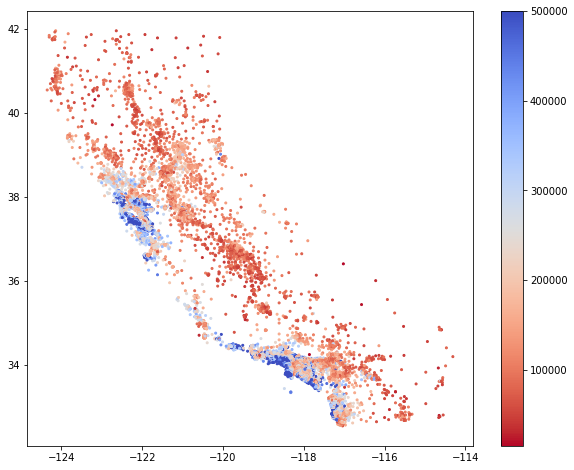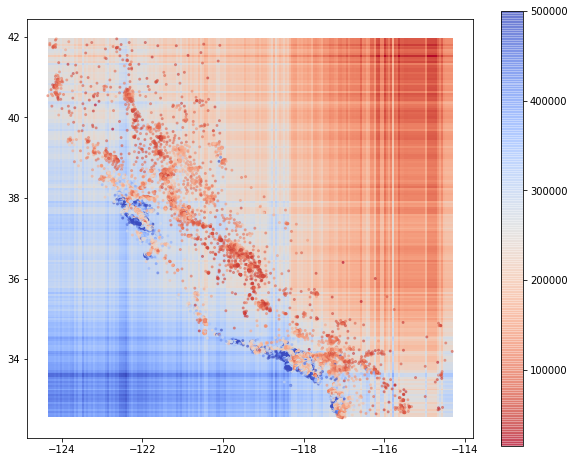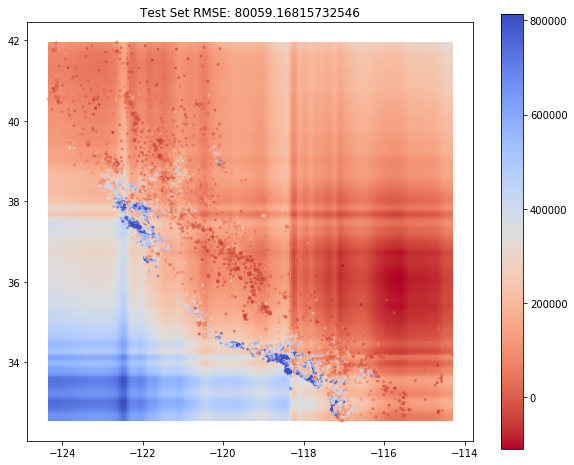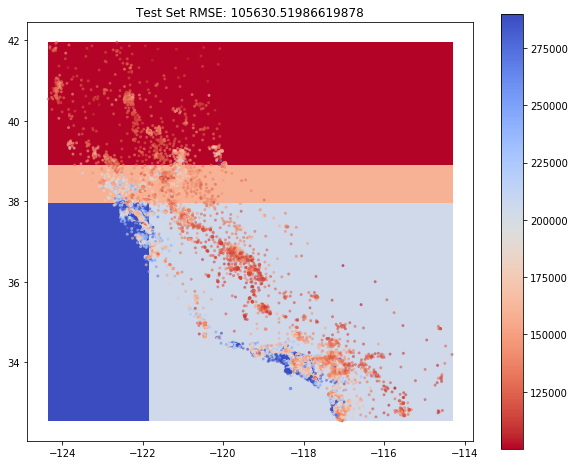### Introduction¶

When it comes to quick out-of-the-box predictive modeling, methods based on Decision Trees are still hand-down one of the easiest to apply yet highly efficient models. Tree ensembles like Random Forests or Boosting achieve high accuracy results without much fine-tuning or feature engineering. One of the drawbacks of common Decision Tree training algorithms is their reliance on a greedy exploration of 'split' space (see e.g. this nice introduction to ID3 and CART). Instead of creating splitting criteria in a manner that results in a final tree with globally lowest error out of all possible trees, we only get optimal splits locally at each node. Despite this little flaw, Decision Tree based algorithms remain one of the most reliable modeling approaches nonetheless. However, it would be cool to try to find a solution to this problem. In this post I want to present an idea that I recently had to potentially solve the issue. At first, I should warn the reader that the current result is neither directly interpretable nor is it a 'valid' Decision Tree, as the implied rules cannot be guaranteed to be mutually exclusive. Nevertheless, I haven't seen this idea before (despite some diligent research effort), so I hope the potential novelty of this idea makes up for this.

### The demonstrative dataset¶

It's always nice to have some data to directly apply an idea to - in this case I chose the well known California Housing prices dataset. Using only the longitude/latitude variables to predict the median house value, this creates a problem that can be nicely visualized to gain some intuition about the underlying concepts.

In :
import pandas as pd
import numpy as np
import itertools

from sklearn.model_selection import train_test_split

from sklearn.linear_model import Lasso, Ridge, ElasticNet

import matplotlib.pyplot as plt

In :
df = pd.read_csv("housing.csv")

In :
df.head()

Out:
longitude latitude housing_median_age total_rooms total_bedrooms population households median_income median_house_value ocean_proximity
0 -122.23 37.88 41.0 880.0 129.0 322.0 126.0 8.3252 452600.0 NEAR BAY
1 -122.22 37.86 21.0 7099.0 1106.0 2401.0 1138.0 8.3014 358500.0 NEAR BAY
2 -122.24 37.85 52.0 1467.0 190.0 496.0 177.0 7.2574 352100.0 NEAR BAY
3 -122.25 37.85 52.0 1274.0 235.0 558.0 219.0 5.6431 341300.0 NEAR BAY
4 -122.25 37.85 52.0 1627.0 280.0 565.0 259.0 3.8462 342200.0 NEAR BAY
In :
df = df[["longitude", "latitude", "median_house_value"]]

X = df.drop("median_house_value",1)
y = pd.DataFrame(df["median_house_value"])

In :
xx = np.ravel(X.values[:,0])
yy = np.ravel(X.values[:,1])
zz = np.ravel(y.values)

plt.figure(figsize = (10,8))
plt.scatter(xx,yy,c=zz,cmap = plt.cm.coolwarm.reversed(), s = 4)
plt.colorbar()

Out:
<matplotlib.colorbar.Colorbar at 0x1a2e5ed7f0>We see that there is some relationship between location and value. Although the relation is not perfect, a general tendency is clearly visible. A standard Decision Tree would definitely need some depth to express the somewhat diagonal line that separates the higher value estates (blue dots) from the lower value estates (red dots).

### Building up the model¶

In my post about the RuleFit algorithm, I showed how a Decision Tree can be expressed as a sum of products of Indicator Functions. A single-node shallow Decision Tree for the Housing dataset could for example look like this:

$$f(x)=\alpha\cdot I_{(longitude > -119)}(x) + \beta\cdot I_{(longitude \leq -119)}(x)\quad\quad(1)$$

Latitudes below $-119$ then indicate a value $\alpha$ and latitudes above $-119$ indicate value $\beta$ respectively. Notice that $(1)$ can also be written as

$$f(x)=\alpha + (\beta-\alpha)\cdot I_{(longitude \leq -119)}(x) = \alpha + \beta^*\cdot I_{(longitude \leq -119)}(x)$$

That means that we can reformulate the simple one node case as a single variable linear model. In a next step, let's expand this to a multiple regression model that uses all possible single splits based on the values of the training data:

$$f(x)=\alpha + \sum_{i=1}^N\beta_{1,i}I_{(longitude \leq longitude(x_i))}(x) + \sum_{i=1}^N\beta_{2,i}I_{(latitude \leq latitude(x_i))}(x)$$

A simple linear regression would clearly overfit here, so I decided to use Lasso for estimation. As long as the amount of non-zero parameter estimates is sufficiently small, we could also fully interpret the rules implied by the linear model like in a Regression Tree.

In the following, I wrote a function that creates the indicators for every training observation over both variables:

In :
class IndicatorCreator:

def fit(self,X):
self.X = np.array(X)
self.X_unique = [np.unique(self.X[:,i]) for i in range(X.shape)]

def transform(self,X):
X = np.array(X)
result_list = []

X_col = 0
for unique_values in self.X_unique:
result_array = np.zeros((X.shape,len(unique_values)))

ar_col = 0
for u_val in unique_values:

result_array[:,ar_col] = (X[:,X_col]<=u_val)
ar_col += 1

result_list.append(result_array.astype(float))
X_col += 1

result = np.concatenate(result_list,1)

return(result)



Let's try that on the dataset:

In :
X_train, X_test, y_train, y_test = train_test_split(X,y, random_state = 42)

indicator_creator = IndicatorCreator()
indicator_creator.fit(X_train)

X_train_t = indicator_creator.transform(X_train)
X_test_t = indicator_creator.transform(X_test)

lasso = Lasso()

lasso.fit(X_train_t,y_train)


Now visualize what the model has learnt:

In :
X_coordinates = np.linspace(X_train.iloc[:,0].min(),X_train.iloc[:,0].max(), 200).reshape(-1,1)
Y_coordinates = np.linspace(X_train.iloc[:,1].min(),X_train.iloc[:,1].max(), 200).reshape(-1,1)

xx_grid, yy_grid = np.meshgrid(X_coordinates, Y_coordinates)

point_space = np.c_[xx_grid.ravel(), yy_grid.ravel()]
indicator_products = indicator_creator.transform(point_space)

preds = lasso.predict(X_test_t).reshape(-1,1)
contour_predictions = lasso.predict(indicator_products).reshape(200,200)

plt.figure(figsize = (10,8))

plt.imshow(contour_predictions, cmap=plt.cm.coolwarm.reversed(), origin='lower',
extent=[np.min(X_coordinates), np.max(X_coordinates), np.min(Y_coordinates), np.max(Y_coordinates)]
)
plt.scatter(xx,yy,c=zz,cmap = plt.cm.coolwarm.reversed(), alpha = .5, s = 4)

plt.colorbar()

Out:
<matplotlib.colorbar.Colorbar at 0x1a1daba5f8>As mentioned earlier, the implicit one-node Decision Tree model doesn't truly capture the non-rectangular split between high value and low value houses. The model simply assigns higher values the more we move to the left and downwards and vice-versa. What is missing is an interaction between longitude and latitude. Only if we move to the lower-left of the map (very roughly) should the house prices actually increase in value. In the indicator framework, this could be achieved via an interaction term like e.g.

$$I_{(longitude \leq -118)}(x)I_{(latitude \leq 38)}(x)$$

Remember that this marks a valid path in a two-noded Decision Tree. To find an optimal decision tree with only two nodes, the Decision Tree algorithm would already have to pick the best decision rules from

$$I_{(longitude \leq longitude(x_i))}(x)I_{(latitude \leq latitude(x_j))}(x)$$

for every combination of $i$ and $j$ and assign corresponding coefficients $\beta$. The search space for the globally best splits will simply explode and thus cannot be fully explored by algorithms that build the model one split at a time. What we need is a method that can potentially explore the full search space at once and find the optimal set of interactions.

Thanks to Kernel Regression, this is actually doable!

### Solving the interaction problem with Kernel Regression¶

In another blog-post, I already wrote about the concept of a Kernel function. What I haven't mentioned there, was that a valid Kernel could be decomposed into another dot-product:

$$K(x,x')=\phi(x)^T\phi(x').$$

In a standard Kernel Regression setting, we perform linear regression on the Gram matrix:

$$f(X)=K(\Phi(X),\Phi(X)')\beta$$

where $\Phi(\cdot)$ denotes rowwise application of $\phi(\cdot)$ on $X$ which is the same matrix $X$ of stacked training input variables $x_i$ as in the standard linear regression setting. Now, the above can be decomposed as

$$f(X)=\Phi(X)\Phi(X')^T\beta = \Phi(X)\beta^*$$

Notice that this last line only happens implicitly since we are actually always working with the Gram-matrix. However, if we find a Kernel $K$ that results in an implicit calculation of all possible indicator interactions of arbitrary depth via $\Phi(X)$, we can implicitly run a linear regression over all possible decision tree rules. Since we want to go with interactions among all single indicators of arbitrary depth, the Kernel of choice is the Polynomial Kernel:

$$K(X,X')=(XX^T+1)^d.$$

where $d\in\mathbb{Z}^+$. By applying this Kernel, we are implicitly calculating all possible $d$ order interactions among all single indicator functions. Since we are only working with indicators, interaction of an indicator with itself is redundant and doesn't affect the outcome; e.g.

$$I_{(\cdot)}(x) ^d = I_{(\cdot)}(x)\quad\forall d\in\mathbb{Z}^+$$

By working out the problem implicity in Hilbert Space, it is possible to implicitly solve the computationally intractable exploration of the full search space. The result however is by no means interpretable as a pure Decision Tree is - maybe there is a way to recover the implicitly applied rules in some way but I am currently not aware of any. However, this approach might open up other technical possibilities as Kernel methods and Hilbert spaces in general are particularly well studied mathematical objects.

Now let's compare the proposed method with a standard Regression Tree:

In :
#Create the Gram Matrices from the indicator features
poly_kernel_train = (X_train_t@np.transpose(X_train_t)/X_train_t.shape+1)**2
poly_kernel_test = (X_test_t@np.transpose(X_train_t)/X_test_t.shape+1)**2

In :
#We use Ridge regression to estimate the regression coefficients
ridge = Ridge()
ridge.fit(poly_kernel_train,y_train)
poly_kernel_preds = ridge2.predict(poly_kernel_test)

rmse_poly = np.sqrt(np.mean((poly_kernel_preds.reshape(-1)-y_test.values.reshape(-1))**2))

In :
kernel_contour = (indicator_products@np.transpose(X_train_t)/X_test_t.shape+1)**2

poly_cont_preds = ridge2.predict(kernel_contour).reshape(200,200)

plt.figure(figsize = (10,8))

plt.imshow(poly_cont_preds, cmap=plt.cm.coolwarm.reversed(), origin='lower',
extent=[np.min(X_coordinates), np.max(X_coordinates), np.min(Y_coordinates), np.max(Y_coordinates)])
plt.colorbar()

plt.scatter(xx,yy,c=zz,cmap = plt.cm.coolwarm.reversed(), alpha = .5, s = 4)
plt.title("Test Set RMSE: "+str(rmse_poly))

Out:
Text(0.5,1,'Test Set RMSE: 80059.16815732546')This looks much nicer than the initial single-node approach. Although it is still not perfect, the model captures the interaction between longitude and altitude much better than before. Now compare that to a Decision Tree, that has an explicit depth of two - notice however that the comparison is somewhat flawed since the decision rules that had been implicitly calculated aren't mutually exclusive. If we were able to translate the implicit indicator representation back to an actual Regression Tree, it would be much deeper than two levels. Focus should be kept more on the technical side and the possibility to explore Decision Trees via Hilbert space theory.

In :
from sklearn.tree import DecisionTreeRegressor

In :
tree = DecisionTreeRegressor(max_depth=2)
tree.fit(X_train,y_train)

preds = tree.predict(X_test)

rmse_tree = np.sqrt(np.mean((preds.reshape(-1)-y_test.values.reshape(-1))**2))

In :
tree_cont_preds = tree.predict(point_space).reshape(200,200)

plt.figure(figsize = (10,8))

plt.imshow(tree_cont_preds, cmap=plt.cm.coolwarm.reversed(), origin='lower',
extent=[np.min(X_coordinates), np.max(X_coordinates), np.min(Y_coordinates), np.max(Y_coordinates)])
plt.colorbar()

plt.scatter(xx,yy,c=zz,cmap = plt.cm.coolwarm.reversed(), alpha = .5, s = 4)
plt.title("Test Set RMSE: "+str(rmse_tree))

Out:
Text(0.5,1,'Test Set RMSE: 105630.51986619878')### Summary and going further¶

In this post we have seen how switching among representations can be helpful to solve problems that are intractable in one representation but totally doable in another. At first, the transition from a graph representation to a representation via indicator functions allowed to explicitly state all possible single node rules in one linear model. By transferring this representation into Hilbert Space it was even possible to implicitly increase search space of possible Decision Rules up to arbitrary size and find search the whole space at once. This would have been impossible with standard CART and similar algorithms. In summary, I really like the idea of leveraging CART algorithms into Hilbert Space and I am eager to explore this approach further. One relatively simple extension would be an ensemble version in the manner of Random Forests or Boosting but I am optimistic that there are much deeper possibilities here.